Courses

# Long Answer Type Questions(Part- 1)- Statistics Class 10 Notes | EduRev

## Class 10 : Long Answer Type Questions(Part- 1)- Statistics Class 10 Notes | EduRev

The document Long Answer Type Questions(Part- 1)- Statistics Class 10 Notes | EduRev is a part of the Class 10 Course Mathematics (Maths) Class 10.
All you need of Class 10 at this link: Class 10

Q1. The median of the following frequency distribution is 35. Find the value of x.

 Class Interval Frequency Cumulativefrequency 0-20 7 7 20-40 8 15 40-60 12 27 60-80 10 37 80-100 8 45 100-120 5 50 Total 50

Also find the modal class.

Sol. Let us prepare the cumulative frequency table:

 Class intervals f cf 0-10 2 2 + 0 = 2 10-20 3 2 + 3 = 5 20-30 x 5 + x = (5 + x) 30-40 6 (5 + x) + 6 = 11 + x 40-50 5 (11 + x) + 5 = 16 + x 50-60 3 (16 + x) + 3 = 19 + x 60-70 2 (19 + x) + 2 = 21 + x Total (21 + x)

Here,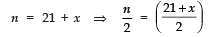Obviously,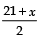lies in the class interval 30−40.

l = 30, Cf = x + 5, f = 6 and h = 10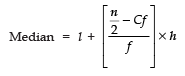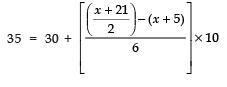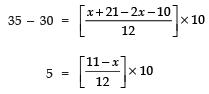⇒ 5 × 12 = 110 − 10x
⇒ 10x = 110 − 60
⇒ 10x =50 ⇒ x = 5

(i) ∴ The required value of x is 5.

(ii) ∵ The maximum frequency is 6
∴ The modal class is 30−40.

Q2. Draw the cumulative frequency curve (ogive) by more than method from the following data:

 Income (in Rs) Number of persons 0-1000 7 1000-2000 15 2000-3000 35 3000-4000 28 4000-5000 10 5000-6000 5

Sol. First, we prepare the cumulative frequency table as follows:

 Income (in Rs) No. of persons Incomemore than Cumulativefrequency 0-1000 7 more than 0 100 - 0 = 100 1000-2000 15 more than 1000 100 - 7 = 93 2000-3000 35 more than 2000 93 - 15 = 78 3000-4000 28 more than 3000 78 - 35 = 43 4000-5000 10 more than 4000 43 - 28 = 15 5000-6000 5 more than 5000 15 - 10 = 5

Thus, we plot the points (0, 100), (1000, 93), (2000, 78), (3000, 43), (4000, 15), (5000, 5) and (6000, 0).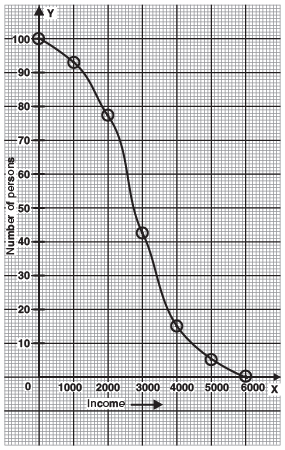Q3. The mean of the following data is 53, find the missing frequencies.

 Age (in years) 0-20 20-40 40-60 60-80 80-100 Total Number of people 15 f 21 f2 17 100

Sol.

 Age (in years) Number of People (fi) Mid value (xi) fi × xi 0-20 15 10 15 x 10 = 150 20-40 fa 30 f1 x 30 = 30 f1 40-60 21 50 21 x 50 = 1050 60-80 f2 70 h x 70 = 70 f2 80-100 17 90 17 x 90 = 1530 Total 100 2730 + 30 f1 + 70 f2

Since, 15 + f1 + 21 + f2 + 17 = 100
∴ 53 + (f1 + f2) = 100

⇒ f1 + f2 = 100 − 53 = 47              ...(1)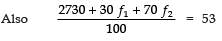⇒ 2730 + 30 f1 + 70 f2 = 5300
⇒ 30 f1 + 70 f2 = 5300 − 2730 = 2570
⇒ 3 f1 + 7 f2 = 257
⇒ 3 (47 − f2) + 7 f2 = 257     |∵ f1 = 47 − f2
⇒ 141 − 3 f2 + 7 f2 = 257
⇒ 4 f2 = 116 or f2 = 29
∴ f1 = 41 − 29 = 18
Thus, f1 = 18 and f2 = 29.

Q4. The mean of the following distribution is 18.

 Class interval 11-13 13-15 15-17 17-19 19-21 21-23 23-25 Frequencies 3 6 9 13 f 5 4

Find f

Sol. Let the assumed mean (a) = 18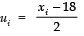∴ We have the following table:

 Class interval xi fi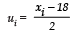fi ui 11-13 12 3 -3 3 x (-3) = -9 13-15 14 6 -2 6 x (-2) = -12 15-17 16 9 -1 9 x (-1) = -9 17-19 18 13 0 13 x 0 = 0 19-21 20 f 1 f x 1 = f 21-23 22 5 2 5 x 2 = 10 23-25 24 4 3 3 x 3 = 9 ∑ fi = 40 + f ∑fi ui = f− 8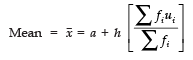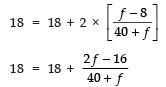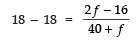⇒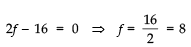Thus, the required frequency = 8

Q5. The percentage of marks obtained by 100 students in an examination are given below:

 Marks 30 - 35 35 - 40 40 - 45 45 - 50 50 -55 55 - 60 60-65 Frequency 14 16 18 23 18 8 3

Find the median of the above data.

Sol.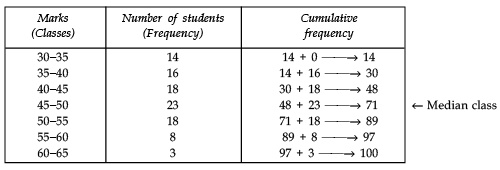Here n = 100 ⇒ n/2 = 50, which lies in the class 45 – 50, where

l1 (lower limit of the median – class) = 45
c (The cumulative frequency of the class preceding the median class) = 48
f (The frequency of the median class) = 23
h (The class size) = 5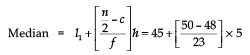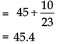∴ The median percentage of marks is 45.4.

Q6. The median of the distribution given below is 14.4. Find the values of x and y, if the total frequency is 20.

 Class interval 0 - 6 6 -12 12 -18 18 - 24 24 - 30 Frequency 4 x 5 y 1

Sol.

 Class interval Frequency CumulativeFrequency 0-6 4 4 + 0 = 4 6-12 x 4 + x = (4 + x) 12-18 5 5 + (4 + x) = 9 + x 18-24 y y + (9 + x) = 9 + x + y 24-30 1 1 + (9 + x + y) = 10 + x + y

Since, n = 20
∴ 10 + x + y = 20
⇒ x + y = 20 − 10
⇒ x + y = 10.      ...(1)

Also, we have
Median = 14.4,
which lies in the class interval 12−18.
∴ The median class is 12−18, such that

l = 12, f = 5, Cf = 4 + x and h = 6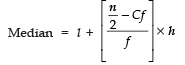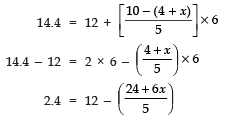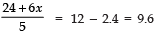⇒ 24 + 6x = (9.6) × 5
⇒ 24 + 6x =48
⇒ 6 x = 48 − 24 = 24

⇒ x = 24/6 = 4

Now, from (1), we have:

x + y = 10
⇒ 4 + y = 10
⇒ y = 10 − 4 = 6
Thus, x =4 and y = 6

Q7. The following table gives production yield per hectare of wheat of 100 farms of a village:

 Production yield (kg/hectare) Number of farms 40-45 4 45-50 6 50-55 16 55-60 20 60-65 30 65-70 24

Change the distribution to a ‘more than type’ distribution, and draw its ogive.

Sol. The given distribution is converted to a “more than type” as:

 Production yield (kg/hectare) Number of farms (cumulative frequencies) More than or equal to 40 100 - 0 = 100 More than or equal to 45 100 - 4 = 96 More than or equal to 50 96 - 6 = 90 More than or equal to 55 90 - 16 = 74 More than or equal to 60 74 - 20 = 54 More than or equal to 65 54 - 30 = 24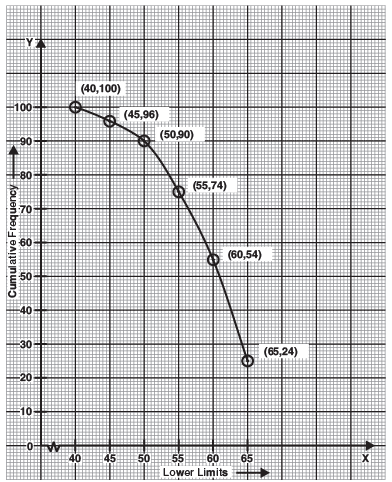Q8. The distribution below gives the weights of 30 students of a class. Find the mean and the median weight of the students:

 Weight (in kg) Number of students 40-45 2 45-50 3 50-55 8 55-60 6 60-65 6 65-70 3 70-75 2

Sol. Let the assumed mean, a = 57.5
∴ h = 5

∴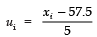We have the following table:

 Weight of students  (in kg) Classmark (xi) Frequencyfi Cumulativefrequency  Cf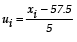fi ui 40-45 42.5 2 2 + 0 = 2 -3 -6 45-50 47.5 3 2 + 3 = 5 -2 -6 50-55 52.5 8 5 + 8 = 13 -1 -8 55-60 57.5 6 13 + 6 = 19 0 0 60-65 62.5 6 19 + 6 = 25 1 6 65-70 67.5 3 25 + 3 = 28 2 6 70-75 72.5 2 28 + 2 = 30 3 6 Total ∑ fi = 30 ∑fiui = - 2

∴ The mean of the given data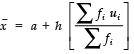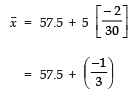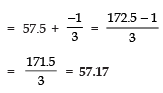For finding the median: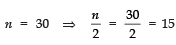And it lies in the class 55–60.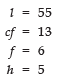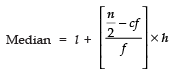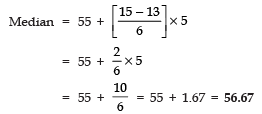Q9. The lengths of 40 leaves of a plant are measured correct upto the nearest millimetre and the data is as under:

 Length (in mm) Numbers of Leaves 118-126 4 126-134 5 134-142 10 142-150 12 150-158 4 158-166 5

Find the mean and median length of the leaves.

Sol. For finding the mean:

Let the assumed mean a = 146
h = 8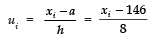Now, we have the following table:

 Length (in mm) Class mark (xi) Frequency (fi) Cumulative frequency Cf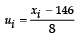fi ui 118-126 122 4 4 + 0= 4 -3 -12 126-134 130 5 4 + 5= 9 -2 -10 134-142 138 10 9 + 10= 19 -1 -10 142-150 146 12 19 + 12= 31 0 0 150-158 154 4 31 + 4= 35 1 4 158-166 162 5 35 + 5= 40 2 10 Total ∑ fi = 40 ∑ fi ui =-18

∴ Mean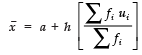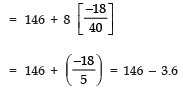= 142.4 mm

Median: Since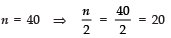[Median class is 142-150]

l = 142
cf = 19
f = 12 and h = 8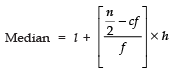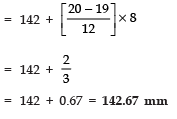Q10. The table below shows the daily expenditure on food of 30 households in a locality:

 Daily expenditure (in Rs) Numbers of households 100-150 6 150-200 7 200-250 12 250-300 3 300-350 2

Find the mean and median daily expenditure on food.

Sol. For finding the mean

Let the assumed mean a = 225.

h = 50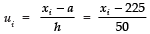We have the following table:

 Daily expenditure xi fi c.f.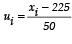fi ui 100-150 125 6 6 + 0 = 6 -2 (-2) x 6 = -12 150-200 175 7 6 + 7 = 13 -1 (-1) x 7 = -7 200-250 225 12 13 + 12 = 25 0 (0) x 12 = 0 250-300 275 3 25 + 3 = 28 1 (1) x 3 = 3 300-350 325 2 28 + 2 = 30 2 (2) x 2 = 4 Total ∑ fi = 30 ∑ fiui =-12

∴ Mean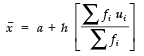⇒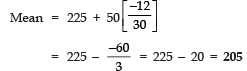To find median: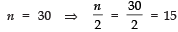And 15 lies in the class 200−250.
∴ Median class is 200−250.

∴ l = 200
cf = 13
f = 12 and h = 50

Thus,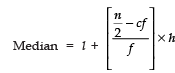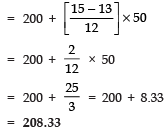Offer running on EduRev: Apply code STAYHOME200 to get INR 200 off on our premium plan EduRev Infinity!

,

,

,

,

,

,

,

,

,

,

,

,

,

,

,

,

,

,

,

,

,

;